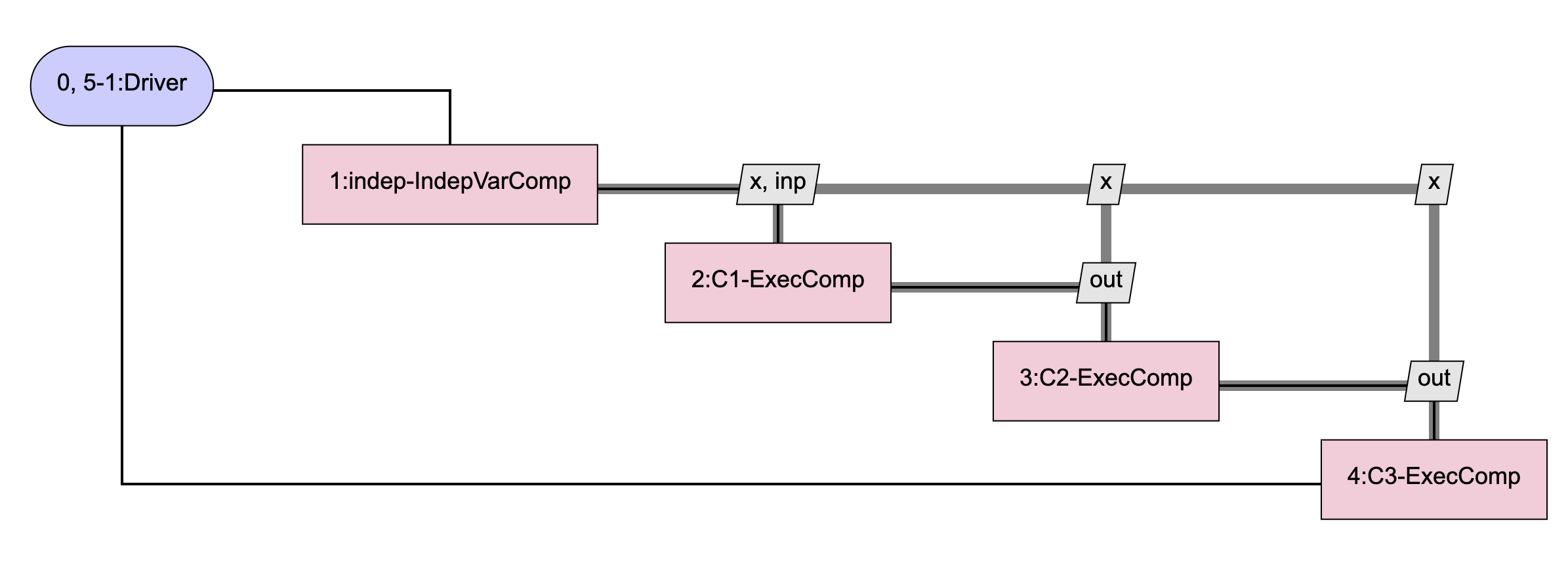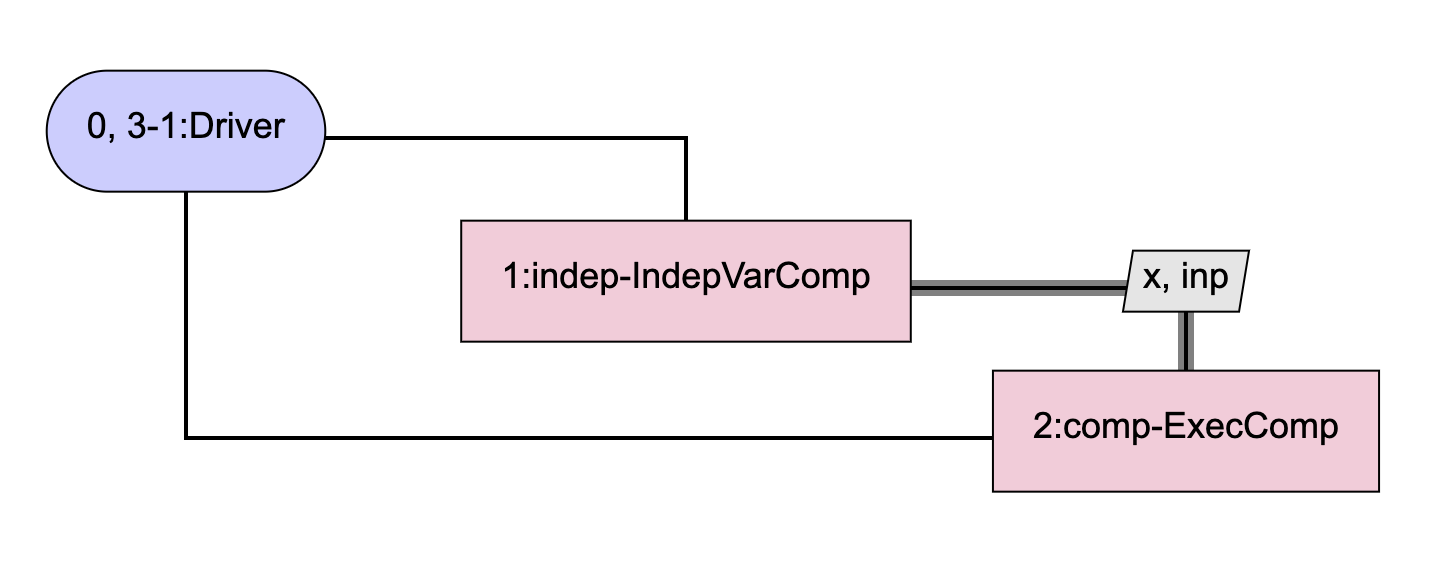# Matrix Free Total Derivatives#

The compute_jacvec_product method of Problem can be used to compute a matrix free total Jacobian vector product. It’s analogous to the way that the compute_jacvec_product method of System can be used to compute partial Jacobian vector products.

Problem.compute_jacvec_product(of, wrt, mode, seed)[source]

Given a seed and ‘of’ and ‘wrt’ variables, compute the total jacobian vector product.

Parameters:
oflist of str

Variables whose derivatives will be computed.

wrtlist of str

Derivatives will be computed with respect to these variables.

modestr

Derivative direction (‘fwd’ or ‘rev’).

seeddict or list

Either a dict keyed by ‘wrt’ varnames (fwd) or ‘of’ varnames (rev), containing dresidual (fwd) or doutput (rev) values, OR a list of dresidual or doutput values that matches the corresponding ‘wrt’ (fwd) or ‘of’ (rev) varname list.

Returns:
dict

The total jacobian vector product, keyed by variable name.

Below is an example of a component that embeds a sub-problem and uses compute_jacvec_product on that sub-problem to compute its Jacobian. The SubProbComp component computes derivatives in both ‘fwd’ and ‘rev’ directions, but in a realistic scenario, it would only compute them in a single direction.

The model that SubProbComp is intended to emulate is one that looks like this:Instead of using 3 identical ExecComps as shown above and having OpenMDAO automatically compute the total derivatives for us, SubProbComp will use just a single ExecComp and will compute its derivatives internally. The model contained in the sub-problem looks like this:The code for SubProbComp is shown below:

import openmdao.api as om

class SubProbComp(om.ExplicitComponent):
"""
This component contains a sub-Problem with a component that will be solved over num_nodes
points instead of creating num_nodes instances of that same component and connecting them
together.
"""
def __init__(self, input_size, num_nodes, mode, **kwargs):
super().__init__(**kwargs)
self.prob = None
self.size = input_size
self.num_nodes = num_nodes
self.mode = mode

def _setup_subprob(self):
self.prob = p = om.Problem(comm=self.comm)
model = self.prob.model

p.setup()
p.final_setup()

def setup(self):
self._setup_subprob()

self.declare_partials('*', '*')

def compute(self, inputs, outputs):
p = self.prob
p['comp.x'] = inputs['x']
p['comp.inp'] = inputs['inp']
inp = inputs['inp']
for i in range(self.num_nodes):
p['comp.inp'] = inp
p.run_model()
inp = p['comp.out']

outputs['out'] = p['comp.out']

def _compute_partials_fwd(self, inputs, partials):
p = self.prob
x = inputs['x']
p['comp.x'] = x
p['comp.inp'] = inputs['inp']

seed = {'comp.x':np.zeros(x.size), 'comp.inp': np.zeros(1)}
p.run_model()
p.model._linearize(None)
for rhsname in seed:
for rhs_i in range(seed[rhsname].size):
seed['comp.x'][:] = 0.0
seed['comp.inp'][:] = 0.0
seed[rhsname][rhs_i] = 1.0
for i in range(self.num_nodes):
p.model._vectors['output']['linear'].set_val(0.0)
p.model._vectors['residual']['linear'].set_val(0.0)
jvp = p.compute_jacvec_product(of=['comp.out'], wrt=['comp.x','comp.inp'], mode='fwd', seed=seed)
seed['comp.inp'][:] = jvp['comp.out']

if rhsname == 'comp.x':
partials[self.pathname + '.out', self.pathname +'.x'][0, rhs_i] = jvp[self.pathname + '.out']
else:
partials[self.pathname + '.out', self.pathname + '.inp'][0, 0] = jvp[self.pathname + '.out']

def _compute_partials_rev(self, inputs, partials):
p = self.prob
p['comp.x'] = inputs['x']
p['comp.inp'] = inputs['inp']
seed = {'comp.out': np.ones(1)}

stack = []
comp = p.model.comp
comp._inputs['inp'] = inputs['inp']
# store the inputs to each comp (the comp at each node point) by doing nonlinear solves
# and storing what the inputs are for each node point.  We'll set these inputs back
# later when we linearize about each node point.
for i in range(self.num_nodes):
stack.append(comp._inputs['inp'])
comp._inputs['x'] = inputs['x']
comp._solve_nonlinear()
comp._inputs['inp'] = comp._outputs['out']

for i in range(self.num_nodes):
p.model._vectors['output']['linear'].set_val(0.0)
p.model._vectors['residual']['linear'].set_val(0.0)
comp._inputs['inp'] = stack.pop()
comp._inputs['x'] = inputs['x']
p.model._linearize(None)
jvp = p.compute_jacvec_product(of=['comp.out'], wrt=['comp.x','comp.inp'], mode='rev', seed=seed)
seed['comp.out'][:] = jvp['comp.inp']

# all of the comp.x's are connected to the same indepvarcomp, so we have
# to accumulate their contributions together
partials[self.pathname + '.out', self.pathname + '.x'] += jvp['comp.x']

# this one doesn't get accumulated because each comp.inp contributes to the
# previous comp's .out (or to comp.inp in the case of the first comp) only.
# Note that we have to handle this explicitly here because normally in OpenMDAO
# we accumulate derivatives when we do reverse transfers.  We can't do that
# here because we only have one instance of our component, so instead of
# accumulating into separate 'comp.out' variables for each comp instance,
# we would be accumulating into a single comp.out variable, which would make
# our derivative too big.
partials[self.pathname + '.out', self.pathname + '.inp'] = jvp['comp.inp']

def compute_partials(self, inputs, partials):
# note that typically you would only have to define partials for one direction,
# either fwd OR rev, not both.
if self.mode == 'fwd':
self._compute_partials_fwd(inputs, partials)
else:
self._compute_partials_rev(inputs, partials)